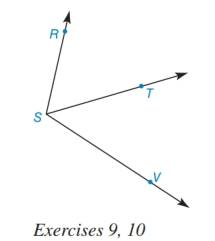Chapter 1.5, Problem 9E### Elementary Geometry for College St...

6th Edition
Daniel C. Alexander + 1 other
ISBN: 9781285195698

#### Solutions

Chapter
Section### Elementary Geometry for College St...

6th Edition
Daniel C. Alexander + 1 other
ISBN: 9781285195698
Textbook Problem
1 views

# In Exercises 7 10, state the property or definition that justifies the conclusion (the “then” clause).Given ∠ R S V and S T → as shown, then m ∠ R S T + m ∠ T S V = m ∠ R S V .To determine

To find:

The property or definition that justifies the conclusion of the statement “Given RSV and ST as shown, then mRST+mTSV=mRSV.”

Explanation

Given:

The given diagram is,

The statement is “Given RSV and ST as shown, then mRST+mTSV=mRSV.”

Approach:

The given statement is based on hypothesis and conclusion. Let RSV and ST shown in the figure be the hypothesis and mRST+mTSV=mRSV be the conclusion

### Still sussing out bartleby?

Check out a sample textbook solution.

See a sample solution

#### The Solution to Your Study Problems

Bartleby provides explanations to thousands of textbook problems written by our experts, many with advanced degrees!

Get Started

#### Find more solutions based on key concepts